## How to make Comparison Matrix

By now you know how to make paired comparisons. In this section you will learn how to make a reciprocal matrix from pair wise comparisons.

For example John has 3 kinds of fruits to be compared and he made subjective judgment on which fruit he likes best, like the following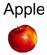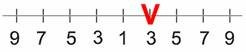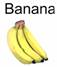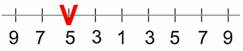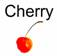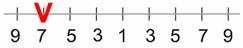We can make a matrix from the 3 comparisons above. Because we have three comparisons, thus we have 3 by 3 matrix. The diagonal elements of the matrix are always 1 and we only need to fill up the upper triangular matrix. How to fill up the upper triangular matrix is using the following rules:

1. If the judgment value is on the left side of 1, we put the actual judgment value.
2. If the judgment value is on the right side of 1, we put the reciprocal value .

Comparing apple and banana, John slightly favor banana, thus we put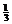in the row 1 column 2 of the matrix. Comparing Apple and Cherry, John strongly likes apple, thus we put actual judgment 5 on the first row, last column of the matrix. Comparing banana and cherry, banana is dominant. Thus we put his actual judgment on the second row, last column of the matrix. Then based on his preference values above, we have a reciprocal matrix like thisTo fill the lower triangular matrix, we use the reciprocal values of the upper diagonal. If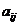is the element of row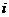column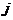of the matrix, then the lower diagonal is filled using this formula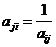Thus now we have complete comparison matrixNotice that all the element in the comparison matrix are positive, or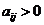.

Next section will discuss about how you will use this matrix.